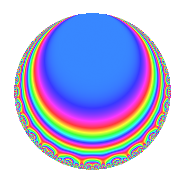# Properties

 Label 189.2.c.cLevel 189 Weight 2 Character orbit 189.c Analytic conductor 1.509 Analytic rank 0 Dimension 4 CM No Inner twists 4

# Learn more about

## Newspace parameters

 Level: $$N$$ = $$189 = 3^{3} \cdot 7$$ Weight: $$k$$ = $$2$$ Character orbit: $$[\chi]$$ = 189.c (of order $$2$$ and degree $$1$$)

## Newform invariants

 Self dual: No Analytic conductor: $$1.5091725982$$ Analytic rank: $$0$$ Dimension: $$4$$ Coefficient field: $$\Q(\sqrt{-2}, \sqrt{3})$$ Coefficient ring: $$\Z[a_1, \ldots, a_{5}]$$ Coefficient ring index: $$2$$ Sato-Tate group: $\mathrm{SU}(2)[C_{2}]$

## $q$-expansion

Coefficients of the $$q$$-expansion are expressed in terms of a basis $$1,\beta_1,\beta_2,\beta_3$$ for the coefficient ring described below. We also show the integral $$q$$-expansion of the trace form.

 $$f(q)$$ $$=$$ $$q$$ $$-\beta_{1} q^{2}$$ $$-\beta_{2} q^{5}$$ $$+ ( 1 - \beta_{3} ) q^{7}$$ $$-2 \beta_{1} q^{8}$$ $$+O(q^{10})$$ $$q$$ $$-\beta_{1} q^{2}$$ $$-\beta_{2} q^{5}$$ $$+ ( 1 - \beta_{3} ) q^{7}$$ $$-2 \beta_{1} q^{8}$$ $$+ \beta_{3} q^{10}$$ $$-\beta_{1} q^{11}$$ $$-\beta_{3} q^{13}$$ $$+ ( -\beta_{1} - 2 \beta_{2} ) q^{14}$$ $$-4 q^{16}$$ $$+ 3 \beta_{2} q^{17}$$ $$+ 3 \beta_{3} q^{19}$$ $$-2 q^{22}$$ $$+ 2 \beta_{1} q^{23}$$ $$-2 q^{25}$$ $$-2 \beta_{2} q^{26}$$ $$+ 5 \beta_{1} q^{29}$$ $$-\beta_{3} q^{31}$$ $$-3 \beta_{3} q^{34}$$ $$+ ( 3 \beta_{1} - \beta_{2} ) q^{35}$$ $$+ 5 q^{37}$$ $$+ 6 \beta_{2} q^{38}$$ $$+ 2 \beta_{3} q^{40}$$ $$+ 5 \beta_{2} q^{41}$$ $$+ 5 q^{43}$$ $$+ 4 q^{46}$$ $$-5 \beta_{2} q^{47}$$ $$+ ( -5 - 2 \beta_{3} ) q^{49}$$ $$+ 2 \beta_{1} q^{50}$$ $$+ 8 \beta_{1} q^{53}$$ $$+ \beta_{3} q^{55}$$ $$+ ( -2 \beta_{1} - 4 \beta_{2} ) q^{56}$$ $$+ 10 q^{58}$$ $$+ 5 \beta_{2} q^{59}$$ $$+ \beta_{3} q^{61}$$ $$-2 \beta_{2} q^{62}$$ $$-8 q^{64}$$ $$+ 3 \beta_{1} q^{65}$$ $$+ 2 q^{67}$$ $$+ ( 6 + \beta_{3} ) q^{70}$$ $$-10 \beta_{1} q^{71}$$ $$-5 \beta_{1} q^{74}$$ $$+ ( -\beta_{1} - 2 \beta_{2} ) q^{77}$$ $$-13 q^{79}$$ $$+ 4 \beta_{2} q^{80}$$ $$-5 \beta_{3} q^{82}$$ $$+ \beta_{2} q^{83}$$ $$-9 q^{85}$$ $$-5 \beta_{1} q^{86}$$ $$-4 q^{88}$$ $$-6 \beta_{2} q^{89}$$ $$+ ( -6 - \beta_{3} ) q^{91}$$ $$+ 5 \beta_{3} q^{94}$$ $$-9 \beta_{1} q^{95}$$ $$+ 7 \beta_{3} q^{97}$$ $$+ ( 5 \beta_{1} - 4 \beta_{2} ) q^{98}$$ $$+O(q^{100})$$ $$\operatorname{Tr}(f)(q)$$ $$=$$ $$4q$$ $$\mathstrut +\mathstrut 4q^{7}$$ $$\mathstrut +\mathstrut O(q^{10})$$ $$4q$$ $$\mathstrut +\mathstrut 4q^{7}$$ $$\mathstrut -\mathstrut 16q^{16}$$ $$\mathstrut -\mathstrut 8q^{22}$$ $$\mathstrut -\mathstrut 8q^{25}$$ $$\mathstrut +\mathstrut 20q^{37}$$ $$\mathstrut +\mathstrut 20q^{43}$$ $$\mathstrut +\mathstrut 16q^{46}$$ $$\mathstrut -\mathstrut 20q^{49}$$ $$\mathstrut +\mathstrut 40q^{58}$$ $$\mathstrut -\mathstrut 32q^{64}$$ $$\mathstrut +\mathstrut 8q^{67}$$ $$\mathstrut +\mathstrut 24q^{70}$$ $$\mathstrut -\mathstrut 52q^{79}$$ $$\mathstrut -\mathstrut 36q^{85}$$ $$\mathstrut -\mathstrut 16q^{88}$$ $$\mathstrut -\mathstrut 24q^{91}$$ $$\mathstrut +\mathstrut O(q^{100})$$

Basis of coefficient ring in terms of a root $$\nu$$ of $$x^{4}\mathstrut +\mathstrut$$ $$4$$ $$x^{2}\mathstrut +\mathstrut$$ $$1$$:

 $$\beta_{0}$$ $$=$$ $$1$$ $$\beta_{1}$$ $$=$$ $$\nu^{3} + 3 \nu$$ $$\beta_{2}$$ $$=$$ $$\nu^{2} + 2$$ $$\beta_{3}$$ $$=$$ $$\nu^{3} + 5 \nu$$
 $$1$$ $$=$$ $$\beta_0$$ $$\nu$$ $$=$$ $$($$$$\beta_{3}\mathstrut -\mathstrut$$ $$\beta_{1}$$$$)/2$$ $$\nu^{2}$$ $$=$$ $$\beta_{2}\mathstrut -\mathstrut$$ $$2$$ $$\nu^{3}$$ $$=$$ $$($$$$-$$$$3$$ $$\beta_{3}\mathstrut +\mathstrut$$ $$5$$ $$\beta_{1}$$$$)/2$$

## Character Values

We give the values of $$\chi$$ on generators for $$\left(\mathbb{Z}/189\mathbb{Z}\right)^\times$$.

 $$n$$ $$29$$ $$136$$ $$\chi(n)$$ $$-1$$ $$-1$$

## Embeddings

For each embedding $$\iota_m$$ of the coefficient field, the values $$\iota_m(a_n)$$ are shown below.

For more information on an embedded modular form you can click on its label.

Label $$\iota_m(\nu)$$ $$a_{2}$$ $$a_{3}$$ $$a_{4}$$ $$a_{5}$$ $$a_{6}$$ $$a_{7}$$ $$a_{8}$$ $$a_{9}$$ $$a_{10}$$
188.1
 0.517638i − 1.93185i − 0.517638i 1.93185i
1.41421i 0 0 −1.73205 0 1.00000 2.44949i 2.82843i 0 2.44949i
188.2 1.41421i 0 0 1.73205 0 1.00000 + 2.44949i 2.82843i 0 2.44949i
188.3 1.41421i 0 0 −1.73205 0 1.00000 + 2.44949i 2.82843i 0 2.44949i
188.4 1.41421i 0 0 1.73205 0 1.00000 2.44949i 2.82843i 0 2.44949i
 $$n$$: e.g. 2-40 or 990-1000 Significant digits: Format: Complex embeddings Normalized embeddings Satake parameters Satake angles

## Inner twists

Char. orbit Parity Mult. Self Twist Proved
1.a Even 1 trivial yes
3.b Odd 1 yes
7.b Odd 1 yes
21.c Even 1 yes

## Hecke kernels

This newform can be constructed as the kernel of the linear operator $$T_{2}^{2}$$ $$\mathstrut +\mathstrut 2$$ acting on $$S_{2}^{\mathrm{new}}(189, [\chi])$$.